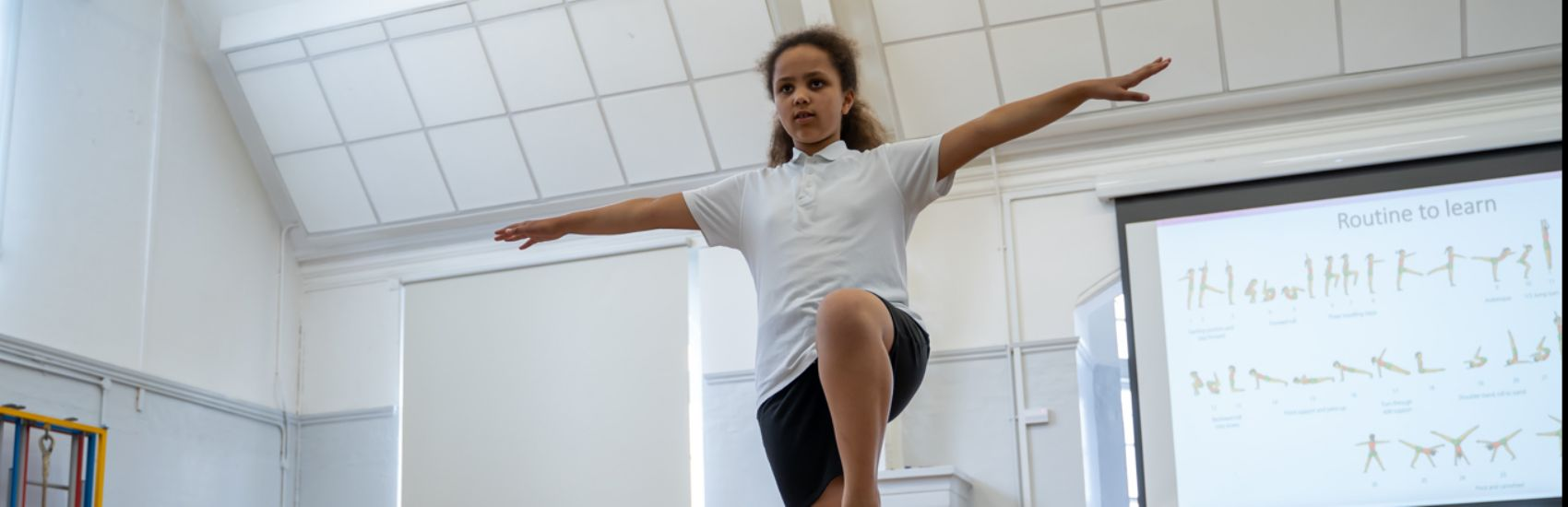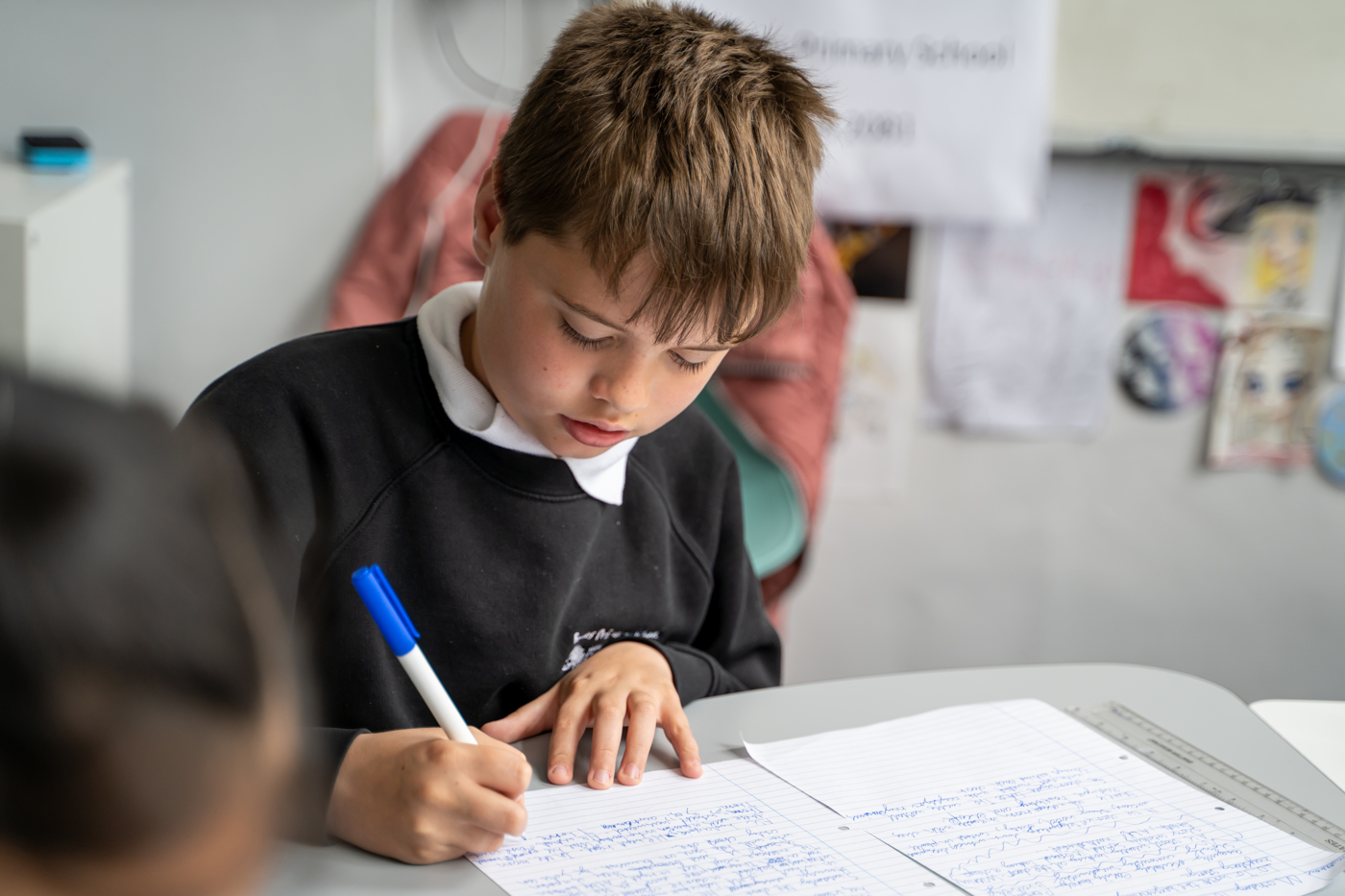''

# Mathematics

Mathematics National Curriculum for Year 3.

Number - number and place value
The children will be taught to:
• count from 0 in multiples of 4, 8, 50 and 100; find 10 or 100 more or less than a given number
• recognise the place value of each digit in a three-digit number (hundreds, tens, ones)
• compare and order numbers up to 1000
• identify, represent and estimate numbers using different representations
• read and write numbers up to 1000 in numerals and in words
• solve number problems and practical problems involving these ideas.
The children will be taught to:
• add and subtract numbers mentally, including:
• a three-digit number and ones
• a three-digit number and tens
• a three-digit number and hundreds
• add and subtract numbers with up to three digits, using formal written methods of columnar addition and subtraction
• estimate the answer to a calculation and use inverse operations to check answers
• solve problems, including missing number problems, using number facts, place value, and more complex addition and subtraction.
Number - multiplication and division
The children will be taught to:
• recall and use multiplication and division facts for the 3, 4 and 8 multiplication tables
• write and calculate mathematical statements for multiplication and division using the multiplication tables that they know, including for two-digit numbers times one-digit numbers, using mental and progressing to formal written methods
• solve problems, including missing number problems, involving multiplication and division, including positive integer scaling problems and correspondence problems in which n objects are connected to m objects.
Number - fractions
The children will be taught to:
• count up and down in tenths; recognise that tenths arise from dividing an object into 10 equal parts and in dividing one-digit numbers or quantities by 10
• recognise, find and write fractions of a discrete set of objects: unit fractions and non-unit fractions with small denominators
• recognise and use fractions as numbers: unit fractions and non-unit fractions with small denominators
• recognise and show, using diagrams, equivalent fractions with small denominators
• add and subtract fractions with the same denominator within one whole
• compare and order unit fractions, and fractions with the same denominators
• solve problems that involve all of the above.
Measurement
The children will be taught to:
• measure, compare, add and subtract: lengths (m/cm/mm); mass (kg/g); volume/capacity (l/ml)
• measure the perimeter of simple 2-D shapes
• add and subtract amounts of money to give change, using both £ and p in practical contexts
• tell and write the time from an analogue clock, including using Roman numerals from I to XII, and 12-hour and 24-hour clocks
• estimate and read time with increasing accuracy to the nearest minute; record and compare time in terms of seconds, minutes and hours; use vocabulary such as o’clock, a.m./p.m., morning, afternoon, noon and midnight
• know the number of seconds in a minute and the number of days in each month, year and leap year
• compare durations of events [for example to calculate the time taken by particular events or tasks].
Geometry - properties of shape
The children will be taught to:
• draw 2-D shapes and make 3-D shapes using modelling materials; recognise 3-D shapes in different orientations and describe them
• recognise angles as a property of shape or a description of a turn
• identify right angles, recognise that two right angles make a half-turn, three make three quarters of a turn and four a complete turn; identify whether angles are greater than or less than a right angle
• identify horizontal and vertical lines and pairs of perpendicular and parallel lines.
Statistics
The children will be taught to:
• interpret and present data using bar charts, pictograms and tables
• solve one-step and two-step questions [for example, ‘How many more?’ and ‘How many fewer?’] using information presented in scaled bar charts and pictograms and tables.